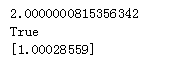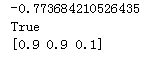• 使用Python非线性数字滤波：对Python在非线性滤波中的应用进行了详细的介绍，是一份值得学习的资料~
• 文章目录非线性样本Sklearn回归汇总决策树随机森林Keras神经网络 非线性样本 from matplotlib import pyplot as mp y = [.4187, .0964, .0853, .0305, .0358, .0338, .0368, .0222, .0798, .1515] x = [[i]for i in...
• 今天小编就为大家分享一篇python 非线性规划方式(scipy.optimize.minimize)，具有很好的参考价值，希望对大家有所帮助。一起跟随小编过来看看吧
• python非线性结构 一. 封装和解构 1.1 封装 将多个用逗号 , 分隔开来的值，组合在一起，形成一个新的元组 t0 = (10,20) t1 = 10,20 x,y = (1,2) print(type(t0)) print(type(t1)) print(&quot;{}+{}={}...
python非线性结构
一. 封装和解构
1.1 封装
将多个用逗号 , 分隔开来的值，组合在一起，形成一个新的元组
t0 = (10,20)
t1 = 10,20
x,y = (1,2)
print(type(t0))
print(type(t1))
print("{}+{}={}".format(x,y,x+y))
x,y = t1
print("{}+{}={}".format(x,y,x+y))
运行结果如下：
<class 'tuple'>
<class 'tuple'>
1+2=3
10+20=30

封装说明
封装:

将多个值使用逗号分割，组合在一起
本质上返回一个元组，只是省略了小括号

1.2.解构
解构就是，把一个list 、tuple 、set 等已经封装起来的类型打开，并且把里面的元素依次赋给标识符。
t = 1, 2, 3 # 这里把这三个元素封装成元组
n1, n2, n3 = t # 把右边元组解构，每个元素依次赋给左边的标识符

n1, n2, n3
(1, 2, 3) # 注意，这里直接用逗号, 连接起来，然后输出，相当于又做了一次封装

print(n1, n2, n3)
1, 2, 3

二.set及操作
python的set和其他语言类似, 是一个无序不重复元素集, 基本功能包括关系测试和消除重复元素. 集合对象还支持union(联合), intersection(交), difference(差)和sysmmetric difference(对称差集)等数学运算.
sets 支持 x in set, len(set),和 for x in set。作为一个无序的集合，sets不记录元素位置或者插入点。因此，sets不支持 indexing, slicing, 或其它类序列（sequence-like）的操作。
2.1.比较
se = {11, 22, 33}
be = {22, 55}
temp1 = se.difference(be)        #找到se中存在，be中不存在的集合，返回新值
print(temp1)        #{33, 11}
print(se)        #{33, 11, 22}

temp2 = se.difference_update(be) #找到se中存在，be中不存在的集合，覆盖掉se
print(temp2)        #None
print(se)           #{33, 11},

2.2.删除
se = {11, 22, 33}
se.discard(11)
se.discard(44)  # 移除不存的元素不会报错
print(se)

se = {11, 22, 33}
se.remove(11)
se.remove(44)  # 移除不存的元素会报错
print(se)

se = {11, 22, 33}  # 移除末尾元素并把移除的元素赋给新值
temp = se.pop()
print(temp)  # 33
print(se) # {11, 22}

2.3.取交集
se = {11, 22, 33}
be = {22, 55}

temp1 = se.intersection(be)             #取交集，赋给新值
print(temp1)  # 22
print(se)  # {11, 22, 33}

temp2 = se.intersection_update(be)      #取交集并更新自己
print(temp2)  # None
print(se)  # 22

2.4.判断
se = {11, 22, 33}
be = {22}

print(se.isdisjoint(be))        #False，判断是否不存在交集（有交集False，无交集True）
print(se.issubset(be))          #False，判断se是否是be的子集合
print(se.issuperset(be))        #True，判断se是否是be的父集合

2.5.合并
se = {11, 22, 33}
be = {22}

temp1 = se.symmetric_difference(be)  # 合并不同项，并赋新值
print(temp1)    #{33, 11}
print(se)       #{33, 11, 22}

temp2 = se.symmetric_difference_update(be)  # 合并不同项，并更新自己
print(temp2)    #None
print(se)             #{33, 11}

2.6.取并集
se = {11, 22, 33}
be = {22,44,55}

temp=se.union(be)   #取并集，并赋新值
print(se)       #{33, 11, 22}
print(temp)     #{33, 22, 55, 11, 44}


三.解析式生成器
返回生成器迭代器的函数。 它看起来像一个普通的函数，只是它包含yield表达式，用于生成一系列可用于for-loop的值，或者可以使用next（）函数一次检索一个值
3.1.生成器的构成：
1.语法格式(返回值 for 元素 in 可迭代对象 if条件)
2.列表解析式的中括号换成小括号即可
3.返回一个生成器
g = ("{:04}".format(i) for i in range(1,6))
print(next(g))
for x in g:
print(x)
print('~~~~~~~~~~~~')
for x in g:
print(x)
运行结果：
0001
0002
0003
0004
0005

3.2.生成器的next不能回头
g = (x for x in range(10) if x % 2)
#取奇数1,3,5,7,9 first=1 second=3 val=4 下一个为5
first = next(g)
second = next(g)
val = first + second
print(val)
print(next(g))
运行结果：
4
5

四.迭代器
迭代器的定义：
表示数据流的对象。 重复调用迭代器的next （）方法（会返回流中的连续项。直到没有数据可以返回时抛出StopIteration异常错误。可以把这个数据流看做一个有序序列，但我们无法提前知道这个序列的长度。同时，Iterator的计算是惰性的，只有通过next()函数时才会计算并返回下一个数据。
from collections import Iterator
print(isinstance("abc",Iterator))
print(isinstance("()",Iterator))
print(isinstance((x for x in range(2)),Iterator))

算法知识补充
一.选择排序法
nums = [1, 9, 8, 5, 6, 7, 4, 3, 2]
length = len(nums)
print(length, ' | ', nums)
count_iter = 0
count_swap = 0

for i in range(length - 1):
maxindex = i
for j in range(i+1, length):
count_iter += 1
if nums[maxindex] < nums[j]:
maxindex = j

if i != maxindex:
nums[i], nums[maxindex] = nums[maxindex], nums[i]
count_swap += 1
print(nums)
print(count_iter, count_swap)

二.优化后的选择排序法（一次循环确定最大值和最小值）
nums = [1, 9, 8, 5, 6, 7, 4, 3, 2]

nums = [1,1,1,1,1,2]

length = len(nums)
print(length, ' | ', nums)
count_iter = 0
count_swap = 0

# 1 9 2
for i in range(length//2): # length - 1
maxindex = i # 假设
minindex = -i - 1 #-1 - i#length - 1 - i

for j in range(i+1, length - i):
count_iter += 1
if nums[maxindex] < nums[j]:
maxindex = j
if nums[minindex] > nums[-j-1]:
minindex = -j - 1 #j

#print("~~~~~", maxindex, minindex)
if nums[maxindex] == nums[minindex]:
break

if i != maxindex:
nums[i], nums[maxindex] = nums[maxindex], nums[i]
count_swap += 1 # 交换过，i对应数应该是最大数
if i - length == minindex: # 交换后，如果有影响，则修正minindex
minindex = maxindex - length

if minindex != -i-1 and nums[minindex] != nums[-i-1]: #and minindex != length - 1 - i:
nums[-i-1], nums[minindex] = nums[minindex], nums[-i-1]
count_swap += 1

print(nums)
print(count_iter, count_swap)

三。求素数
按照定义，从1 开始 到 n-1都没有找到整除它的数，就是质数
import datetime

start = datetime.datetime.now()
n = 100000
#print(2)
count = 1

for x in range(3, n, 2):
for i in range(3, int(x**0.5)+1, 2):
if x % i == 0:
break
else:
count += 1
#print(x)

delta = (datetime.datetime.now() - start).total_seconds()
print(count)
print(delta)
print('-' * 30)

孪生素数
 5 孪生素数
import datetime

start = datetime.datetime.now()
n = 100000
#print(2, 3, 5)
count = 3

x[j+1] = a + 1

x = 7
step = 4
while x < n:
if x % 5 != 0:
#print(x) # 测试是不是质数
for i in range(3, int(x**0.5)+1, 2):
if x % i == 0:
break
else:
count += 1
#print(x)

x += step # 7
step = 4 if step==2 else 2

delta = (datetime.datetime.now() - start).total_seconds()
print(count)
print(delta)
print('-' * 30)

求转置矩阵
lst=[[1,2,3],[4,5,6]]
mn= [[0 for i in range(len(lst))] for j in range (len(lst))]
for  i,a in enumerate(lst):
for j,b in enumerate(a):
mn[j][i]=lst[i][j]
print(mn)


感谢大家支持：
欢迎志同道合的朋友：成为小灰灰的朋友展开全文算法
• 背景：现在项目上有一个用python 实现非线性规划的需求。非线性规划可以简单分两种，目标函数为凸函数 or 非凸函数。 凸函数的 非线性规划，比如fun=x^2+y^2+x*y，有很多常用的python库来完成，网上也有很多资料，...
一.背景：现在项目上有一个用python 实现非线性规划的需求。非线性规划可以简单分两种，目标函数为凸函数 or 非凸函数。

凸函数的 非线性规划，比如fun=x^2+y^2+x*y，有很多常用的python库来完成，网上也有很多资料，比如CVXPY

非凸函数的 非线性规划（求极值），从处理方法来说，可以尝试以下几种：

1.纯数学方法，求导求极值；

2.使用神经网络，深度学习来处理，可参考反向传播算法中链式求导的过程；

3.寻找一些python库来做，本文介绍scipy.optimize.minimize的使用方法

二.库方法介绍

官方文档：https://docs.scipy.org/doc/scipy/reference/generated/scipy.optimize.minimize.html

来看下改方法的入参

scipy.optimize.minimize(fun, x0, args=(), method=None, jac=None, hess=None, hessp=None, bounds=None, constraints=(), tol=None, callback=None, options=None)

解释：

fun: 求最小值的目标函数

x0:变量的初始猜测值，如果有多个变量，需要给每个变量一个初始猜测值。minimize是局部最优的解法，所以

args:常数值,后面demo会讲解，fun中没有数字，都以变量的形式表示，对于常数项，需要在这里给值

method:求极值的方法，官方文档给了很多种。一般使用默认。每种方法我理解是计算误差，反向传播的方式不同而已，这块有很大理论研究空间

constraints:约束条件，针对fun中为参数的部分进行约束限制

三.demo

1.计算 1/x+x 的最小值

# coding=utf-8
from scipy.optimize import minimize
import numpy as np

#demo 1
#计算 1/x+x 的最小值
def fun(args):
a=args
v=lambda x:a/x +x
return v

if __name__ == "__main__":
args = (1)  #a
x0 = np.asarray((2))  # 初始猜测值
res = minimize(fun(args), x0, method='SLSQP')
print(res.fun)
print(res.success)
print(res.x)

执行结果:函数的最小值为2点多，可以看出minimize求的局部最优2.计算  (2+x1)/(1+x2) - 3*x1+4*x3 的最小值  x1,x2,x3的范围都在0.1到0.9 之间

# coding=utf-8
from scipy.optimize import minimize
import numpy as np

# demo 2
#计算  (2+x1)/(1+x2) - 3*x1+4*x3 的最小值  x1,x2,x3的范围都在0.1到0.9 之间
def fun(args):
a,b,c,d=args
v=lambda x: (a+x)/(b+x) -c*x+d*x
return v
def con(args):
# 约束条件 分为eq 和ineq
#eq表示 函数结果等于0 ； ineq 表示 表达式大于等于0
x1min, x1max, x2min, x2max,x3min,x3max = args
cons = ({'type': 'ineq', 'fun': lambda x: x - x1min},\
{'type': 'ineq', 'fun': lambda x: -x + x1max},\
{'type': 'ineq', 'fun': lambda x: x - x2min},\
{'type': 'ineq', 'fun': lambda x: -x + x2max},\
{'type': 'ineq', 'fun': lambda x: x - x3min},\
{'type': 'ineq', 'fun': lambda x: -x + x3max})
return cons

if __name__ == "__main__":
#定义常量值
args = (2,1,3,4)  #a,b,c,d
#设置参数范围/约束条件
args1 = (0.1,0.9,0.1, 0.9,0.1,0.9)  #x1min, x1max, x2min, x2max
cons = con(args1)
#设置初始猜测值
x0 = np.asarray((0.5,0.5,0.5))

res = minimize(fun(args), x0, method='SLSQP',constraints=cons)
print(res.fun)
print(res.success)
print(res.x)

执行结果：对于这种简单的函数，可以看出局部最优的求解和真实最优解相差不大，对于复杂的函数，x0的初始值设置，会很大程度影响最优解的结果。

ADD:

全局最优的函数： scipy.optimize.basinhopping

有一个缺点是无法设置约束，求全局的最优解的函数

https://docs.scipy.org/doc/scipy/reference/generated/scipy.optimize.basinhopping.html
展开全文scipy
• python 非线性规划（from scipy.optimize import minimize）库方法介绍拓展 库方法介绍 官方文档：https://docs.scipy.org/doc/scipy/reference/generated/scipy.optimize.minimize.html 方法的入参: scipy....
python 非线性规划（from scipy.optimize import minimize）库方法介绍拓展
库方法介绍
官方文档：https://docs.scipy.org/doc/scipy/reference/generated/scipy.optimize.minimize.html

方法的入参:
scipy.optimize.minimize(fun, x0, args=(), method=None, jac=None, hess=None, hessp=None, bounds=None, constraints=(), tol=None, callback=None, options=None)

fun:求最小值的目标函数
x0:变量的初始猜测值，如果有多个变量，需要给每个变量一个初始猜测值。
args:常数值，fun中没有数字，都以变量的形式表示，对于常数项，需要在这里给值
method:求极值的方法，官方文档给了很多种。一般使用默认。每种方法我理解是计算误差，反向传播的方式不同而已，这块有很大理论研究空间
constraints:约束条件，针对fun中为参数的部分进行约束限制
from scipy.optimize import minimize

# 计算 1/x+x 的最小值
def fun(x):
return 1 / x + x

if __name__ == "__main__":
x0 = 2 #初始猜测值
res = minimize(fun, x0, method='SLSQP')
print(res.fun)
print(res.success)
print(res.x)

“”“
执行结果
2.0000000815356342
True
[1.00028559]
“””


力扣：5463. 服务中心的最佳位置https://leetcode-cn.com/problems/best-position-for-a-service-centre/
一家快递公司希望在新城市建立新的服务中心。公司统计了该城市所有客户在二维地图上的坐标，并希望能够以此为依据为新的服务中心选址：使服务中心 到所有客户的欧几里得距离的总和最小 。
给你一个数组 positions ，其中 positions[i] = [xi, yi] 表示第 i 个客户在二维地图上的位置，返回到所有客户的 欧几里得距离的最小总和
提示：
1 <= positions.length <= 50
positions[i].length == 2
0 <= positions[i], positions[i] <= 100

from scipy.optimize import minimize
class Solution:
def getMinDistSum(self, positions: List[List[int]]) -> float:
def getSum(x):
return sum(sqrt((x - x1) ** 2 + (x - y1) ** 2 ) for x1, y1 in positions)
return minimize(getSum, [50,50]).fun

拓展
全局最优的函数： scipy.optimize.basinhopping
有一个缺点是无法设置约束，求全局的最优解的函数
官方文档：
https://docs.scipy.org/doc/scipy/reference/generated/scipy.optimize.basinhopping.html


展开全文算法 数据分析 人工智能
• 非线性规划问题分为两种问题：无约束和约束。 不受约束的优化问题是形式上的问题 min⁡f(x),x=[x1,x2,⋯ ,xn]T∈Rn  (1)\min \boldsymbol{f}\left( \boldsymbol{x} \right) ,\boldsymbol{x}=\left[ \boldsymbol{x...
Python      MATLAB
MATLAB和Python全文档
不受约束的问题
非线性规划问题分为两种问题：无约束和约束。 不受约束的优化问题是形式上的问题
$\min \boldsymbol{f}\left( \boldsymbol{x} \right) ,\boldsymbol{x}=\left[ \boldsymbol{x}_1,\boldsymbol{x}_2,\cdots ,\boldsymbol{x}_{\boldsymbol{n}} \right] ^{\boldsymbol{T}}\in \mathbb{R}^{\boldsymbol{n}}\,\, \left( 1 \right)$
一个一般的约束优化问题包括方程（1）中具有相等约束的目标函数：
$\boldsymbol{E}\left( \boldsymbol{x} \right) =0 \left( 2 \right)$
和不平等约束：
$\boldsymbol{I}\left( \boldsymbol{x} \right) \leqslant 0 \left( 3 \right)$
其中，$\boldsymbol{x}\in \mathbb{R}^{\boldsymbol{n}},\boldsymbol{E}\left( \boldsymbol{x} \right) \in \mathbb{R}^{\boldsymbol{p}}和\,\,\boldsymbol{I}\left( \boldsymbol{x} \right) \in \mathbb{R}^{\boldsymbol{q}}$
在本节中，将考虑上述问题（1）。如果$\boldsymbol{x}^*=\left[ \boldsymbol{x}_{1}^{*},\boldsymbol{x}_{2}^{*},\cdots ,\boldsymbol{x}_{\boldsymbol{n}}^{*} \right] ^{\boldsymbol{T}}\in \mathbb{R}^{\boldsymbol{n}}$是问题的最优解，则
$\boldsymbol{g}\left( \boldsymbol{x}^* \right) =0 \left( 4 \right) \\\boldsymbol{y}^{\boldsymbol{T}}\boldsymbol{H}\left( \boldsymbol{x}^* \right) \boldsymbol{y}\geqslant 0,\forall \boldsymbol{y}\in \mathbb{R}^{\boldsymbol{n}}\,\, \left( 5 \right)$
其中$\boldsymbol{g}\left( \boldsymbol{x} \right) =\nabla \boldsymbol{f}\left( \boldsymbol{x} \right)$ 是梯度向量，$\boldsymbol{H}\left( \boldsymbol{x} \right)$ 是由下式定义的黑森矩阵,
$\boldsymbol{g}\left( \boldsymbol{x} \right) =\nabla \boldsymbol{f}\left( \boldsymbol{x} \right) =\left[ \begin{array}{c} \frac{\partial \boldsymbol{f}\left( \boldsymbol{x} \right)}{\partial \boldsymbol{x}_1}\\ \frac{\partial \boldsymbol{f}\left( \boldsymbol{x} \right)}{\boldsymbol{x}_2}\\ \vdots\\ \frac{\partial \boldsymbol{f}\left( \boldsymbol{x} \right)}{\partial \boldsymbol{x}_{\boldsymbol{n}}}\\\end{array} \right] \\\boldsymbol{H}\left( \boldsymbol{x} \right) =\left[ \begin{matrix} \frac{\partial ^2\boldsymbol{f}\left( \boldsymbol{x} \right)}{\partial \boldsymbol{x}_{1}^{2}}& \frac{\partial ^2\boldsymbol{f}\left( \boldsymbol{x} \right)}{\partial \boldsymbol{x}_1\partial \boldsymbol{x}_2}& \cdots& \frac{\partial ^2\boldsymbol{f}\left( \boldsymbol{x} \right)}{\partial \boldsymbol{x}_1\partial \boldsymbol{x}_{\boldsymbol{n}}}\\ \frac{\partial ^2\boldsymbol{f}\left( \boldsymbol{x} \right)}{\partial \boldsymbol{x}_2\partial \boldsymbol{x}_1}& \frac{\partial ^2\boldsymbol{f}\left( \boldsymbol{x} \right)}{\partial \boldsymbol{x}_{2}^{2}}& \cdots& \frac{\partial ^2\boldsymbol{f}\left( \boldsymbol{x} \right)}{\partial \boldsymbol{x}_2\partial \boldsymbol{x}_{\boldsymbol{n}}}\\ \vdots& \vdots& \ddots& \vdots\\ \frac{\partial ^2\boldsymbol{f}\left( \boldsymbol{x} \right)}{\partial \boldsymbol{x}_{\boldsymbol{n}}\partial \boldsymbol{x}_1}& \frac{\partial ^2\boldsymbol{f}\left( \boldsymbol{x} \right)}{\partial \boldsymbol{x}_{\boldsymbol{n}}\partial \boldsymbol{x}_2}& \cdots& \frac{\partial ^2\boldsymbol{f}\left( \boldsymbol{x} \right)}{\partial \boldsymbol{x}_{\boldsymbol{n}}^{2}}\\\end{matrix} \right]$
等式（5）表示黑森矩阵是一个正半定数。 式（4）-（5）是$\boldsymbol{x}^*$的必要最优条件。 如果条件（5）替换为条件：
$\boldsymbol{y}^{\boldsymbol{T}}\boldsymbol{H}\left( \boldsymbol{x}* \right) \boldsymbol{y}>0,\forall \boldsymbol{y}\in \mathbb{R}^{\boldsymbol{n}}\,\, \left( 6 \right)$
（即，黑森矩阵是正定的），则条件（4）-（6）是充分的最优性条件。
用于求解（1）的数值方法是迭代的。 他们将从解$\boldsymbol{x}^*$的初始猜测$\boldsymbol{x}^{\left( 0 \right)}$开始，然后构造解$\boldsymbol{x}^{\left( 0 \right)},\boldsymbol{x}^{\left( 1 \right)},\boldsymbol{x}^{\left( 2 \right)},\cdots ,$的序列，其中$\boldsymbol{f}\left( \boldsymbol{x}^{\left( 0 \right)} \right) >\boldsymbol{f}\left( \boldsymbol{x}^{\left( 1 \right)} \right) >\cdots$ 在迭代$\boldsymbol{k}$时，数值优化中从点$\boldsymbol{x}^{\left( \boldsymbol{k} \right)}$移至点$\boldsymbol{x}^{\left( \boldsymbol{k}+1 \right)}$通常需要经历两个步骤：第一步是搜索方向$\boldsymbol{p}^{\left( \boldsymbol{k} \right)}$确定，然后在第二步中沿$\boldsymbol{p}^{\left( \boldsymbol{k} \right)}$方向进行线搜索（其中$\left\| \boldsymbol{p}^{\left( \boldsymbol{k}+1 \right)} \right\| =1$）以找到最小点$\boldsymbol{x}^{\left( \boldsymbol{k}+1 \right)}=\boldsymbol{x}^{\left( \boldsymbol{k} \right)}+\boldsymbol{\alpha p}^{\left( \boldsymbol{k} \right)}$，使得$\boldsymbol{f}\left( \boldsymbol{x}^{\left( \boldsymbol{k}+1 \right)} \right) <\boldsymbol{f}\left( \boldsymbol{x}^{\left( \boldsymbol{k} \right)} \right)$ 。 如果满足以下条件，则迭代过程在解$\boldsymbol{x}^{\left( \boldsymbol{k} \right)}$处停止。
$\left\| \boldsymbol{g}\left( \boldsymbol{x}^{\left( \boldsymbol{k} \right)} \right) \right\| <\boldsymbol{\varepsilon }$
其中$\boldsymbol{\varepsilon }<0$是任意小的正实数，而$\boldsymbol{H}\left( \boldsymbol{x}^{\left( \boldsymbol{k} \right)} \right)$ 是正半定数。
无约束优化的数值优化技术关注两个问题：

线搜索问题：给定一个函数$\boldsymbol{f}\left( \boldsymbol{x} \right)$ ，其梯度$\boldsymbol{g}\left( \boldsymbol{x} \right) =\nabla \boldsymbol{f}\left( \boldsymbol{x} \right)$ ，在迭代$\boldsymbol{k}$下降方向$\boldsymbol{p}^{\left( \boldsymbol{k} \right)}$上的一个点$\boldsymbol{x}^{\left( \boldsymbol{k} \right)}$，找到$\boldsymbol{\alpha }^{\left( \boldsymbol{k} \right)}>0$使得对于$\boldsymbol{\alpha }=\boldsymbol{\alpha }^{\left( \boldsymbol{k} \right)}$，沿着射线$\boldsymbol{x}^{\left( \boldsymbol{k} \right)}+\boldsymbol{\alpha p}^{\left( \boldsymbol{k} \right)}$函数$\boldsymbol{f}$最小化，即
$\boldsymbol{\alpha }^{\left( \boldsymbol{k} \right)}=\boldsymbol{arg}\underset{\boldsymbol{\alpha }>0}{\min}\left\{ \boldsymbol{f}\left( \boldsymbol{x}^{\left( \boldsymbol{k} \right)}+\boldsymbol{\alpha p}^{\left( \boldsymbol{k} \right)} \right) \right\}$

搜索方向问题：给定一个函数$\boldsymbol{f}\left( \boldsymbol{x} \right)$ 和点$\boldsymbol{x}^{\left( \boldsymbol{k} \right)}$，在迭代$\boldsymbol{k}$处，找到一个单位矢量$\boldsymbol{p}^{\left( \boldsymbol{k} \right)}$，使得$\boldsymbol{f}\left( \boldsymbol{x}^{\left( \boldsymbol{k} \right)}+\boldsymbol{\alpha p}^{\left( \boldsymbol{k} \right)} \right)$ 对于$0<\boldsymbol{\alpha }<\boldsymbol{\alpha }_{\max}$是递减函数。 即$\boldsymbol{p}^{\left( \boldsymbol{k} \right)}$是下降方向。

数值优化方法的不同之处在于确定搜索方向以及对梯度矢量和黑森矩阵进行近似和（或）更新的方法。
线搜索算法
线搜索算法有两种：精确线搜索和不精确线搜索算法。 精确线搜索算法会寻找精确步长$\boldsymbol{\alpha }\left( \boldsymbol{\alpha }=\boldsymbol{\alpha }_{\boldsymbol{Exact}} \right)$ ，以便
$\boldsymbol{\alpha }_{\boldsymbol{Exact}}=\boldsymbol{arg}\underset{\boldsymbol{\alpha }>0}{\min}\left\{ \boldsymbol{f}\left( \boldsymbol{x}^{\left( \boldsymbol{k} \right)}+\boldsymbol{\alpha p}^{\left( \boldsymbol{k} \right)} \right) \right\}$
在数字上不切实际。 不精确的线搜索算法寻找与精确步长$\boldsymbol{\alpha }_{\boldsymbol{Exact}}$近似的值。 从初始猜测$\boldsymbol{\alpha }^0$开始，逐步构建步长序列$\boldsymbol{\alpha }^0,\boldsymbol{\alpha }^1,\boldsymbol{\alpha }^2,\cdots ,\boldsymbol{\alpha }^{\boldsymbol{k}}$的序列，以使$\boldsymbol{\alpha }^{\boldsymbol{k}}\approx \boldsymbol{\alpha }_{\boldsymbol{Exact}}$迭代地完成。
最著名的不精确线搜索算法是回溯线搜索算法，该算法使用两个参数$\boldsymbol{a}$和$\boldsymbol{b}$并基于Armijo条件：
$\boldsymbol{f}\left( \boldsymbol{x}^{\left( \boldsymbol{k} \right)}+\boldsymbol{\alpha }^{\boldsymbol{k}}\boldsymbol{p}^{\left( \boldsymbol{k} \right)} \right) <\boldsymbol{f}\left( \boldsymbol{x} \right) +\boldsymbol{a\alpha }^{\boldsymbol{k}}\boldsymbol{g}\left( \boldsymbol{x}^{\left( \boldsymbol{k} \right)} \right) ^{\boldsymbol{T}}\boldsymbol{p}^{\left( \boldsymbol{k} \right)}$
参数$\boldsymbol{a}$与Armijo的终止条件相关。 参数$0<\boldsymbol{b}<1$用于在每次迭代中更新步长，其中$\boldsymbol{\alpha }^{\boldsymbol{j}}=\boldsymbol{b\alpha }^{\boldsymbol{j}-1}$，从大步长$\boldsymbol{\alpha }^0$（通常= 1）开始。
MATLAB函数LineSearch.m接收一个函数$\boldsymbol{f}$，代表起始向量$\boldsymbol{x}$的$\boldsymbol{f}$梯度的向量$\boldsymbol{g}$和单位（方向）向量$\boldsymbol{p}$并返回最佳步长$\boldsymbol{\alpha }$：
function alpha = LineSearch(f, g, x, p)
a = 0.3 ; b = 0.9 ;
alpha = 1.0 ;
while f(x+alpha*p) > f(x) + a*alpha*g(x)'*p
alpha = b*alpha ;
end
end

函数LineSearch.py的Python代码是：
import numpy as np
def LineSearch(f, g, x, p):
a, b = 0.3, 0.9
alpha = 1.0
while f(x+alpha*p) > f(x) + a*alpha*np.dot(g(x), p):
alpha *= b
return alpha

最陡下降法
牛顿法
拟牛顿法
用MATLAB解决无约束的优化问题
用Gekko解决无约束的优化问题
解决约束的优化问题
MATLAB fmincon函数解决约束优化问题
Python解决约束最小化问题
Gekko Python解决约束优化
详情参阅http://viadean.com/matlab_python_nonlinear_prg.html


展开全文matlab
• 一.封装与解构 1.封装(装箱) a.将多个值使用逗号分割,组合在一起 b.本质上,返回一个元组,只是省掉了小括号 t1 = (1,2)#定义为元组 t2 = 1,2 #将1和2封装成元组 type(t1) ...a.把线性结构的...
• ## Python非线性方程组

千次阅读 2017-07-14 23:08:14
from scipy.optimize import fsolve from math import cos def f(x): d = 140 l = 156 a = float(x) r = float(x) return [ cos(a) - 1 + (d*d)/(2*r*r), #方程1
• 本篇针对非线性规（优）划（化）问题，介绍两个好用的模型构建不求解工具：CppAD与pyomo 。这两个工具都可以只构建目标函数与约束函数的形式，而不需要求其雅可比、海森矩阵。两个工具都可以接受非线性模型形式，...
• # scipy.optimize.minimize(fun, x0, args=(), method=None, jac=None, hess=None, hessp=None, bounds=None, constraints=(), tol=None, callback=None, options=None) # 解释： # fun: 求最小值的目标函数 ...
• 系统的真实模型都是非线性，在实际模型构建或使用过程中，我们会对模型进行线性化。例如，我们根据已有的模型求解最优控制问题：让机器人根据当前的状态输出最优的动作，从而使成本函数取最小值。常见的优化库都需要...pyomo CppAD 自动微分
• kears搭建简单的非线性回归，博主用它进行的武汉市疫情确诊、疑似、死亡、治愈人数的预测。 # -*- encoding: utf-8 -*- """ @File : test.py @Time : 2020/5/27 9:26 @Author : ligang @WeChat : 15188607997 @...tensorflow 神经网络 深度学习 逻辑回归...

# python非线性python 订阅